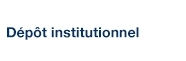# Generalized Linear Mixed Models for Binary Data: Are Matching Results from Penalized Quasi-Likelihood and Numerical Integration Less Biased?

Chowell, Gerardo; Benedetti, Andrea; Platt, Robert et Atherton, Juli (2014). « Generalized Linear Mixed Models for Binary Data: Are Matching Results from Penalized Quasi-Likelihood and Numerical Integration Less Biased? ». PLoS ONE, 9(1), e84601.

Fichier(s) associé(s) à ce document :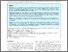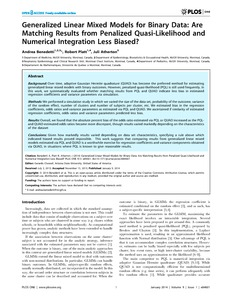Prévisualisation
PDF
Télécharger (1MB)

## Résumé

Background: Over time, adaptive Gaussian Hermite quadrature (QUAD) has become the preferred method for estimating generalized linear mixed models with binary outcomes. However, penalized quasi-likelihood (PQL) is still used frequently. In this work, we systematically evaluated whether matching results from PQL and QUAD indicate less bias in estimated regression coefficients and variance parameters via simulation. Methods: We performed a simulation study in which we varied the size of the data set, probability of the outcome, variance of the random effect, number of clusters and number of subjects per cluster, etc. We estimated bias in the regression coefficients, odds ratios and variance parameters as estimated via PQL and QUAD. We ascertained if similarity of estimated regression coefficients, odds ratios and variance parameters predicted less bias. Results: Overall, we found that the absolute percent bias of the odds ratio estimated via PQL or QUAD increased as the PQLand QUAD-estimated odds ratios became more discrepant, though results varied markedly depending on the characteristics of the dataset Conclusions: Given how markedly results varied depending on data set characteristics, specifying a rule above which indicated biased results proved impossible. This work suggests that comparing results from generalized linear mixed models estimated via PQL and QUAD is a worthwhile exercise for regression coefficients and variance components obtained via QUAD, in situations where PQL is known to give reasonable results.

Type: Article de revue scientifique adaptive Gaussian Hermite quadrature (QUAD), penalized quasi-likelihood (PQL), simulation study Faculté des sciences > Département de mathématiques Juli Atherton 02 mai 2016 19:10 19 mai 2016 18:54 http://archipel.uqam.ca/id/eprint/8397

## Statistiques

Voir les statistiques sur cinq ans...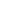RECHERCHER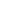PARCOURIR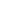LIBRE ACCÈS